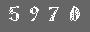# 在应用中嵌入Python

翻译: gashero

# 1   高层次嵌入

```#include <Python.h>
int
main(int argc, char* argv[]) {
Py_Initialize();
PyRun_SimpleString("from time import time,ctime\n"
"print 'Today is',ctime(time())\n");
Py_Finalize();
return 0;
}```

# 2   超越高层嵌入：预览

1. 转换Python类型到C类型
2. 传递参数并调用C函数
3. 转换返回值到Python

1. 转换C数据到Python
2. 调用Python接口程序来调用Python函数
3. 转化返回值到C

# 3   纯扩展

```#include <Python.h>

int
main(int argc, char *argv[])
{
PyObject *pName, *pModule, *pDict, *pFunc;
PyObject *pArgs, *pValue;
int i;

if (argc < 3) {
fprintf(stderr,"Usage: call pythonfile funcname [args]\n");
return 1;
}

Py_Initialize();
pName = PyString_FromString(argv);
/* Error checking of pName left out */

pModule = PyImport_Import(pName);
Py_DECREF(pName);

if (pModule != NULL) {
pFunc = PyObject_GetAttrString(pModule, argv);
/* pFunc is a new reference */

if (pFunc && PyCallable_Check(pFunc)) {
pArgs = PyTuple_New(argc - 3);
for (i = 0; i < argc - 3; ++i) {
pValue = PyInt_FromLong(atoi(argv[i + 3]));
if (!pValue) {
Py_DECREF(pArgs);
Py_DECREF(pModule);
fprintf(stderr, "Cannot convert argument\n");
return 1;
}
/* pValue reference stolen here: */
PyTuple_SetItem(pArgs, i, pValue);
}
pValue = PyObject_CallObject(pFunc, pArgs);
Py_DECREF(pArgs);
if (pValue != NULL) {
printf("Result of call: %ld\n", PyInt_AsLong(pValue));
Py_DECREF(pValue);
}
else {
Py_DECREF(pFunc);
Py_DECREF(pModule);
PyErr_Print();
fprintf(stderr,"Call failed\n");
return 1;
}
}
else {
if (PyErr_Occurred())
PyErr_Print();
fprintf(stderr, "Cannot find function \"%s\"\n", argv);
}
Py_XDECREF(pFunc);
Py_DECREF(pModule);
}
else {
PyErr_Print();
fprintf(stderr, "Failed to load \"%s\"\n", argv);
return 1;
}
Py_Finalize();
return 0;
}```

```def multiply(a,b):
print "Will compute",a,"times",b
c=0
for i in range(0,a)
c=c+b
return c```

```\$ call multiply multiply 3 2
Will compute 3 times 2
Result of call: 6```

```Py_Initialize();
pName=PyString_FromString(argv);
/* Error checking of pName left out */
pModule=PyImport_Import(pName);```

```pFunc=PyObject_GetAttrString(pModule,argv);
/* pFunc is a new reference */
if (pFunc && PyCallable_Check(pFunc)) {
...
}
Py_XDECREF(pFunc);```

`pValue=PyObject_CallObject(pFunc,pArgs);`

# 4   扩展嵌入的Python

```static int numargs=0;

/* Return the number of arguments of the application command line */
static PyObject*
emb_numargs(PyObject *self, PyObject *args)
{
if(!PyArg_ParseTuple(args, ":numargs"))
return NULL;
return Py_BuildValue("i", numargs);
}

static PyMethodDef EmbMethods[] = {
{"numargs", emb_numargs, METH_VARARGS,
"Return the number of arguments received by the process."},
{NULL, NULL, 0, NULL}
};```

```numargs=argc;
Py_InitModule("emb",EmbMethods);```

```import emb
print "Number of arguments",emb.numargs()```

# 6   链接必备条件

configure 脚本执行时，可以正确的生成动态链接库使用的导出符号，而这些却不会自动被嵌入的静态链接的Python所继承，至少是在Unix。这是用于静态链接运行库(libpython.a)并且需要载入动态扩展(.so)的方式。

```>>> import distutils.sysconfig